Intermediate Algebra

# 6.2Factor Trinomials

Intermediate Algebra6.2 Factor Trinomials

### Learning Objectives

By the end of this section, you will be able to:
• Factor trinomials of the form $x2+bx+cx2+bx+c$
• Factor trinomials of the form $ax2+bx+cax2+bx+c$ using trial and error
• Factor trinomials of the form $ax2+bx+cax2+bx+c$ using the ‘ac’ method
• Factor using substitution

### Be Prepared 6.2

Before you get started, take this readiness quiz.

1. Find all the factors of 72.
If you missed this problem, review Example 1.2.
2. Find the product: $(3y+4)(2y+5).(3y+4)(2y+5).$
If you missed this problem, review Example 5.28.
3. Simplify: $−9(6);−9(6);$ $−9(−6).−9(−6).$
If you missed this problem, review Example 1.18.

### Factor Trinomials of the Form $x2+bx+cx2+bx+c$

You have already learned how to multiply binomials using FOIL. Now you’ll need to “undo” this multiplication. To factor the trinomial means to start with the product, and end with the factors.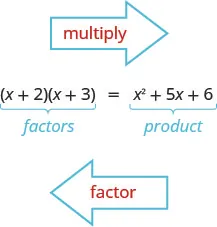To figure out how we would factor a trinomial of the form $x2+bx+c,x2+bx+c,$ such as $x2+5x+6x2+5x+6$ and factor it to $(x+2)(x+3),(x+2)(x+3),$ let’s start with two general binomials of the form $(x+m)(x+m)$ and $(x+n).(x+n).$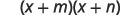Foil to find the product.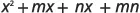Factor the GCF from the middle terms.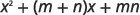Our trinomial is of the form $x2+bx+c.x2+bx+c.$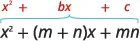This tells us that to factor a trinomial of the form $x2+bx+c,x2+bx+c,$ we need two factors $(x+m)(x+m)$ and $(x+n)(x+n)$ where the two numbers m and n multiply to c and add to b.

### Example 6.9

#### How to Factor a Trinomial of the form $x2+bx+cx2+bx+c$

Factor: $x2+11x+24.x2+11x+24.$

### Try It 6.17

Factor: $q2+10q+24.q2+10q+24.$

### Try It 6.18

Factor: $t2+14t+24.t2+14t+24.$

Let’s summarize the steps we used to find the factors.

### How To

#### Factor trinomials of the form $x2+bx+c.x2+bx+c.$

1. Step 1. Write the factors as two binomials with first terms x. $x2+bx+c(x)(x)x2+bx+c(x)(x)$
2. Step 2.
Find two numbers m and n that
• multiply to $c,m·n=cc,m·n=c$
• add to $b,m+n=bb,m+n=b$
3. Step 3. Use m and n as the last terms of the factors. $(x+m)(x+n)(x+m)(x+n)$
4. Step 4. Check by multiplying the factors.

In the first example, all terms in the trinomial were positive. What happens when there are negative terms? Well, it depends which term is negative. Let’s look first at trinomials with only the middle term negative.

How do you get a positive product and a negative sum? We use two negative numbers.

### Example 6.10

Factor: $y2−11y+28.y2−11y+28.$

### Try It 6.19

Factor: $u2−9u+18.u2−9u+18.$

### Try It 6.20

Factor: $y2−16y+63.y2−16y+63.$

Now, what if the last term in the trinomial is negative? Think about FOIL. The last term is the product of the last terms in the two binomials. A negative product results from multiplying two numbers with opposite signs. You have to be very careful to choose factors to make sure you get the correct sign for the middle term, too.

How do you get a negative product and a positive sum? We use one positive and one negative number.

When we factor trinomials, we must have the terms written in descending order—in order from highest degree to lowest degree.

### Example 6.11

Factor: $2x+x2−48.2x+x2−48.$

### Try It 6.21

Factor: $9m+m2+18.9m+m2+18.$

### Try It 6.22

Factor: $−7n+12+n2.−7n+12+n2.$

Sometimes you’ll need to factor trinomials of the form $x2+bxy+cy2x2+bxy+cy2$ with two variables, such as $x2+12xy+36y2.x2+12xy+36y2.$ The first term, $x2,x2,$ is the product of the first terms of the binomial factors, $x·x.x·x.$ The $y2y2$ in the last term means that the second terms of the binomial factors must each contain y. To get the coefficients b and c, you use the same process summarized in How To Factor trinomials.

### Example 6.12

Factor: $r2−8rs−9s2.r2−8rs−9s2.$

### Try It 6.23

Factor: $a2−11ab+10b2.a2−11ab+10b2.$

### Try It 6.24

Factor: $m2−13mn+12n2.m2−13mn+12n2.$

Some trinomials are prime. The only way to be certain a trinomial is prime is to list all the possibilities and show that none of them work.

### Example 6.13

Factor: $u2−9uv−12v2.u2−9uv−12v2.$

### Try It 6.25

Factor: $x2−7xy−10y2.x2−7xy−10y2.$

### Try It 6.26

Factor: $p2+15pq+20q2.p2+15pq+20q2.$

Let’s summarize the method we just developed to factor trinomials of the form $x2+bx+c.x2+bx+c.$

### Strategy for Factoring Trinomials of the Form $x 2 + b x + c x 2 + b x + c$

When we factor a trinomial, we look at the signs of its terms first to determine the signs of the binomial factors.

$x2+bx+c(x+m)(x+n)Whencis positive,mandnhave the same sign.bpositivebnegativem,npositivem,nnegativex2+5x+6x2−6x+8(x+2)(x+3)(x−4)(x−2)same signssame signsWhencis negative,mandnhave opposite signs.x2+x−12x2−2x−15(x+4)(x−3)(x−5)(x+3)opposite signsopposite signsx2+bx+c(x+m)(x+n)Whencis positive,mandnhave the same sign.bpositivebnegativem,npositivem,nnegativex2+5x+6x2−6x+8(x+2)(x+3)(x−4)(x−2)same signssame signsWhencis negative,mandnhave opposite signs.x2+x−12x2−2x−15(x+4)(x−3)(x−5)(x+3)opposite signsopposite signs$

Notice that, in the case when m and n have opposite signs, the sign of the one with the larger absolute value matches the sign of b.

### Factor Trinomials of the form ax2 + bx + c using Trial and Error

Our next step is to factor trinomials whose leading coefficient is not 1, trinomials of the form $ax2+bx+c.ax2+bx+c.$

Remember to always check for a GCF first! Sometimes, after you factor the GCF, the leading coefficient of the trinomial becomes 1 and you can factor it by the methods we’ve used so far. Let’s do an example to see how this works.

### Example 6.14

Factor completely: $4x3+16x2−20x.4x3+16x2−20x.$

### Try It 6.27

Factor completely: $5x3+15x2−20x.5x3+15x2−20x.$

### Try It 6.28

Factor completely: $6y3+18y2−60y.6y3+18y2−60y.$

What happens when the leading coefficient is not 1 and there is no GCF? There are several methods that can be used to factor these trinomials. First we will use the Trial and Error method.

Let’s factor the trinomial $3x2+5x+2.3x2+5x+2.$

From our earlier work, we expect this will factor into two binomials.

$3x2+5x+2()()3x2+5x+2()()$

We know the first terms of the binomial factors will multiply to give us $3x2.3x2.$ The only factors of $3x23x2$ are $1x,3x.1x,3x.$ We can place them in the binomials.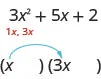Check: Does $1x·3x=3x2?1x·3x=3x2?$

We know the last terms of the binomials will multiply to 2. Since this trinomial has all positive terms, we only need to consider positive factors. The only factors of 2 are 1, 2. But we now have two cases to consider as it will make a difference if we write 1, 2 or 2, 1.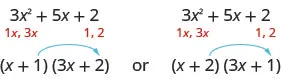Which factors are correct? To decide that, we multiply the inner and outer terms.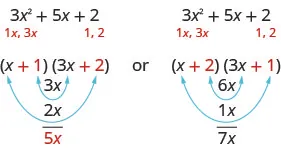Since the middle term of the trinomial is $5x,5x,$ the factors in the first case will work. Let’s use FOIL to check.

$(x+1)(3x+2)3x2+2x+3x+23x2+5x+2✓(x+1)(3x+2)3x2+2x+3x+23x2+5x+2✓$

Our result of the factoring is:

$3x2+5x+2(x+1)(3x+2)3x2+5x+2(x+1)(3x+2)$

### Example 6.15

#### How to Factor a Trinomial Using Trial and Error

Factor completely using trial and error: $3y2+22y+7.3y2+22y+7.$

### Try It 6.29

Factor completely using trial and error: $2a2+5a+3.2a2+5a+3.$

### Try It 6.30

Factor completely using trial and error: $4b2+5b+1.4b2+5b+1.$

### How To

#### Factor trinomials of the form $ax2+bx+cax2+bx+c$ using trial and error.

1. Step 1. Write the trinomial in descending order of degrees as needed.
2. Step 2. Factor any GCF.
3. Step 3. Find all the factor pairs of the first term.
4. Step 4. Find all the factor pairs of the third term.
5. Step 5. Test all the possible combinations of the factors until the correct product is found.
6. Step 6. Check by multiplying.

Remember, when the middle term is negative and the last term is positive, the signs in the binomials must both be negative.

### Example 6.16

Factor completely using trial and error: $6b2−13b+5.6b2−13b+5.$

### Try It 6.31

Factor completely using trial and error: $8x2−14x+3.8x2−14x+3.$

### Try It 6.32

Factor completely using trial and error: $10y2−37y+7.10y2−37y+7.$

When we factor an expression, we always look for a greatest common factor first. If the expression does not have a greatest common factor, there cannot be one in its factors either. This may help us eliminate some of the possible factor combinations.

### Example 6.17

Factor completely using trial and error: $18x2−37xy+15y2.18x2−37xy+15y2.$

### Try It 6.33

Factor completely using trial and error $18x2−3xy−10y2.18x2−3xy−10y2.$

### Try It 6.34

Factor completely using trial and error: $30x2−53xy−21y2.30x2−53xy−21y2.$

Don’t forget to look for a GCF first and remember if the leading coefficient is negative, so is the GCF.

### Example 6.18

Factor completely using trial and error: $−10y4−55y3−60y2.−10y4−55y3−60y2.$

### Try It 6.35

Factor completely using trial and error: $15n3−85n2+100n.15n3−85n2+100n.$

### Try It 6.36

Factor completely using trial and error: $56q3+320q2−96q.56q3+320q2−96q.$

### Factor Trinomials of the Form $ax2+bx+cax2+bx+c$ using the “ac” Method

Another way to factor trinomials of the form $ax2+bx+cax2+bx+c$ is the “ac” method. (The “ac” method is sometimes called the grouping method.) The “ac” method is actually an extension of the methods you used in the last section to factor trinomials with leading coefficient one. This method is very structured (that is step-by-step), and it always works!

### Example 6.19

#### How to Factor Trinomials using the “ac” Method

Factor using the ‘ac’ method: $6x2+7x+2.6x2+7x+2.$

### Try It 6.37

Factor using the ‘ac’ method: $6x2+13x+2.6x2+13x+2.$

### Try It 6.38

Factor using the ‘ac’ method: $4y2+8y+3.4y2+8y+3.$

The “ac” method is summarized here.

### How To

#### Factor trinomials of the form $ax2+bx+cax2+bx+c$ using the “ac” method.

1. Step 1. Factor any GCF.
2. Step 2. Find the product ac.
3. Step 3. Find two numbers m and n that:
$Multiply toacm·n=a·cAdd tobm+n=bax2+bx+cMultiply toacm·n=a·cAdd tobm+n=bax2+bx+c$
4. Step 4. Split the middle term using m and n. $ax2+mx+nx+cax2+mx+nx+c$
5. Step 5. Factor by grouping.
6. Step 6. Check by multiplying the factors.

Don’t forget to look for a common factor!

### Example 6.20

Factor using the ‘ac’ method: $10y2−55y+70.10y2−55y+70.$

### Try It 6.39

Factor using the ‘ac’ method: $16x2−32x+12.16x2−32x+12.$

### Try It 6.40

Factor using the ‘ac’ method: $18w2−39w+18.18w2−39w+18.$

### Factor Using Substitution

Sometimes a trinomial does not appear to be in the $ax2+bx+cax2+bx+c$ form. However, we can often make a thoughtful substitution that will allow us to make it fit the $ax2+bx+cax2+bx+c$ form. This is called factoring by substitution. It is standard to use u for the substitution.

In the $ax2+bx+c,ax2+bx+c,$ the middle term has a variable, x, and its square, $x2,x2,$ is the variable part of the first term. Look for this relationship as you try to find a substitution.

### Example 6.21

Factor by substitution: $x4−4x2−5.x4−4x2−5.$

### Try It 6.41

Factor by substitution: $h4+4h2−12.h4+4h2−12.$

### Try It 6.42

Factor by substitution: $y4−y2−20.y4−y2−20.$

Sometimes the expression to be substituted is not a monomial.

### Example 6.22

Factor by substitution: $(x−2)2+7(x−2)+12(x−2)2+7(x−2)+12$

### Try It 6.43

Factor by substitution: $(x−5)2+6(x−5)+8.(x−5)2+6(x−5)+8.$

### Try It 6.44

Factor by substitution: $(y−4)2+8(y−4)+15.(y−4)2+8(y−4)+15.$

### Media

Access this online resource for additional instruction and practice with factoring.

### Section 6.2 Exercises

#### Practice Makes Perfect

Factor Trinomials of the Form $x2+bx+cx2+bx+c$

In the following exercises, factor each trinomial of the form $x2+bx+c.x2+bx+c.$

61.

$p 2 + 11 p + 30 p 2 + 11 p + 30$

62.

$w 2 + 10 x + 21 w 2 + 10 x + 21$

63.

$n 2 + 19 n + 48 n 2 + 19 n + 48$

64.

$b 2 + 14 b + 48 b 2 + 14 b + 48$

65.

$a 2 + 25 a + 100 a 2 + 25 a + 100$

66.

$u 2 + 101 u + 100 u 2 + 101 u + 100$

67.

$x 2 − 8 x + 12 x 2 − 8 x + 12$

68.

$q 2 − 13 q + 36 q 2 − 13 q + 36$

69.

$y 2 − 18 y − 45 y 2 − 18 y − 45$

70.

$m 2 − 13 m + 30 m 2 − 13 m + 30$

71.

$x 2 − 8 x + 7 x 2 − 8 x + 7$

72.

$y 2 − 5 y + 6 y 2 − 5 y + 6$

73.

$5 p − 6 + p 2 5 p − 6 + p 2$

74.

$6 n − 7 + n 2 6 n − 7 + n 2$

75.

$8 − 6 x + x 2 8 − 6 x + x 2$

76.

$7 x + x 2 + 6 7 x + x 2 + 6$

77.

$x 2 − 12 − 11 x x 2 − 12 − 11 x$

78.

$−11 − 10 x + x 2 −11 − 10 x + x 2$

In the following exercises, factor each trinomial of the form $x2+bxy+cy2.x2+bxy+cy2.$

79.

$x 2 − 2 x y − 80 y 2 x 2 − 2 x y − 80 y 2$

80.

$p 2 − 8 p q − 65 q 2 p 2 − 8 p q − 65 q 2$

81.

$m 2 − 64 m n − 65 n 2 m 2 − 64 m n − 65 n 2$

82.

$p 2 − 2 p q − 35 q 2 p 2 − 2 p q − 35 q 2$

83.

$a 2 + 5 a b − 24 b 2 a 2 + 5 a b − 24 b 2$

84.

$r 2 + 3 r s − 28 s 2 r 2 + 3 r s − 28 s 2$

85.

$x 2 − 3 x y − 14 y 2 x 2 − 3 x y − 14 y 2$

86.

$u 2 − 8 u v − 24 v 2 u 2 − 8 u v − 24 v 2$

87.

$m 2 − 5 m n + 30 n 2 m 2 − 5 m n + 30 n 2$

88.

$c 2 − 7 c d + 18 d 2 c 2 − 7 c d + 18 d 2$

Factor Trinomials of the Form $ax2+bx+cax2+bx+c$ Using Trial and Error

In the following exercises, factor completely using trial and error.

89.

$p 3 − 8 p 2 − 20 p p 3 − 8 p 2 − 20 p$

90.

$q 3 − 5 q 2 − 24 q q 3 − 5 q 2 − 24 q$

91.

$3 m 3 − 21 m 2 + 30 m 3 m 3 − 21 m 2 + 30 m$

92.

$11 n 3 − 55 n 2 + 44 n 11 n 3 − 55 n 2 + 44 n$

93.

$5 x 4 + 10 x 3 − 75 x 2 5 x 4 + 10 x 3 − 75 x 2$

94.

$6 y 4 + 12 y 3 − 48 y 2 6 y 4 + 12 y 3 − 48 y 2$

95.

$2 t 2 + 7 t + 5 2 t 2 + 7 t + 5$

96.

$5 y 2 + 16 y + 11 5 y 2 + 16 y + 11$

97.

$11 x 2 + 34 x + 3 11 x 2 + 34 x + 3$

98.

$7 b 2 + 50 b + 7 7 b 2 + 50 b + 7$

99.

$4 w 2 − 5 w + 1 4 w 2 − 5 w + 1$

100.

$5 x 2 − 17 x + 6 5 x 2 − 17 x + 6$

101.

$4 q 2 − 7 q − 2 4 q 2 − 7 q − 2$

102.

$10 y 2 − 53 y − 11 10 y 2 − 53 y − 11$

103.

$6 p 2 − 19 p q + 10 q 2 6 p 2 − 19 p q + 10 q 2$

104.

$21 m 2 − 29 m n + 10 n 2 21 m 2 − 29 m n + 10 n 2$

105.

$4 a 2 + 17 a b − 15 b 2 4 a 2 + 17 a b − 15 b 2$

106.

$6 u 2 + 5 u v − 14 v 2 6 u 2 + 5 u v − 14 v 2$

107.

$−16 x 2 − 32 x − 16 −16 x 2 − 32 x − 16$

108.

$−81 a 2 + 153 a + 18 −81 a 2 + 153 a + 18$

109.

$−30 q 3 − 140 q 2 − 80 q −30 q 3 − 140 q 2 − 80 q$

110.

$−5 y 3 − 30 y 2 + 35 y −5 y 3 − 30 y 2 + 35 y$

Factor Trinomials of the Form $ax2+bx+cax2+bx+c$ using the ‘ac’ Method

In the following exercises, factor using the ‘ac’ method.

111.

$5 n 2 + 21 n + 4 5 n 2 + 21 n + 4$

112.

$8 w 2 + 25 w + 3 8 w 2 + 25 w + 3$

113.

$4 k 2 − 16 k + 15 4 k 2 − 16 k + 15$

114.

$5 s 2 − 9 s + 4 5 s 2 − 9 s + 4$

115.

$6 y 2 + y − 15 6 y 2 + y − 15$

116.

$6 p 2 + p − 22 6 p 2 + p − 22$

117.

$2 n 2 − 27 n − 45 2 n 2 − 27 n − 45$

118.

$12 z 2 − 41 z − 11 12 z 2 − 41 z − 11$

119.

$60 y 2 + 290 y − 50 60 y 2 + 290 y − 50$

120.

$6 u 2 − 46 u − 16 6 u 2 − 46 u − 16$

121.

$48 z 3 − 102 z 2 − 45 z 48 z 3 − 102 z 2 − 45 z$

122.

$90 n 3 + 42 n 2 − 216 n 90 n 3 + 42 n 2 − 216 n$

123.

$16 s 2 + 40 s + 24 16 s 2 + 40 s + 24$

124.

$24 p 2 + 160 p + 96 24 p 2 + 160 p + 96$

125.

$48 y 2 + 12 y − 36 48 y 2 + 12 y − 36$

126.

$30 x 2 + 105 x − 60 30 x 2 + 105 x − 60$

Factor Using Substitution

In the following exercises, factor using substitution.

127.

$x 4 − x 2 − 12 x 4 − x 2 − 12$

128.

$x 4 + 2 x 2 − 8 x 4 + 2 x 2 − 8$

129.

$x 4 − 3 x 2 − 28 x 4 − 3 x 2 − 28$

130.

$x 4 − 13 x 2 − 30 x 4 − 13 x 2 − 30$

131.

$( x − 3 ) 2 − 5 ( x − 3 ) − 36 ( x − 3 ) 2 − 5 ( x − 3 ) − 36$

132.

$( x − 2 ) 2 − 3 ( x − 2 ) − 54 ( x − 2 ) 2 − 3 ( x − 2 ) − 54$

133.

$( 3 y − 2 ) 2 − ( 3 y − 2 ) − 2 ( 3 y − 2 ) 2 − ( 3 y − 2 ) − 2$

134.

$( 5 y − 1 ) 2 − 3 ( 5 y − 1 ) − 18 ( 5 y − 1 ) 2 − 3 ( 5 y − 1 ) − 18$

Mixed Practice

In the following exercises, factor each expression using any method.

135.

$u 2 − 12 u + 36 u 2 − 12 u + 36$

136.

$x 2 − 14 x − 32 x 2 − 14 x − 32$

137.

$r 2 − 20 r s + 64 s 2 r 2 − 20 r s + 64 s 2$

138.

$q 2 − 29 q r − 96 r 2 q 2 − 29 q r − 96 r 2$

139.

$12 y 2 − 29 y + 14 12 y 2 − 29 y + 14$

140.

$12 x 2 + 36 y − 24 z 12 x 2 + 36 y − 24 z$

141.

$6 n 2 + 5 n − 4 6 n 2 + 5 n − 4$

142.

$3 q 2 + 6 q + 2 3 q 2 + 6 q + 2$

143.

$13 z 2 + 39 z − 26 13 z 2 + 39 z − 26$

144.

$5 r 2 + 25 r + 30 5 r 2 + 25 r + 30$

145.

$3 p 2 + 21 p 3 p 2 + 21 p$

146.

$7 x 2 − 21 x 7 x 2 − 21 x$

147.

$6 r 2 + 30 r + 36 6 r 2 + 30 r + 36$

148.

$18 m 2 + 15 m + 3 18 m 2 + 15 m + 3$

149.

$24 n 2 + 20 n + 4 24 n 2 + 20 n + 4$

150.

$4 a 2 + 5 a + 2 4 a 2 + 5 a + 2$

151.

$x 4 − 4 x 2 − 12 x 4 − 4 x 2 − 12$

152.

$x 4 − 7 x 2 − 8 x 4 − 7 x 2 − 8$

153.

$( x + 3 ) 2 − 9 ( x + 3 ) − 36 ( x + 3 ) 2 − 9 ( x + 3 ) − 36$

154.

$( x + 2 ) 2 − 25 ( x + 2 ) − 54 ( x + 2 ) 2 − 25 ( x + 2 ) − 54$

#### Writing Exercises

155.

Many trinomials of the form $x2+bx+cx2+bx+c$ factor into the product of two binomials $(x+m)(x+n).(x+m)(x+n).$ Explain how you find the values of m and n.

156.

Tommy factored $x2−x−20x2−x−20$ as $(x+5)(x−4).(x+5)(x−4).$ Sara factored it as $(x+4)(x−5).(x+4)(x−5).$ Ernesto factored it as $(x−5)(x−4).(x−5)(x−4).$ Who is correct? Explain why the other two are wrong.

157.

List, in order, all the steps you take when using the “ac” method to factor a trinomial of the form $ax2+bx+c.ax2+bx+c.$

158.

How is the “ac” method similar to the “undo FOIL” method? How is it different?

#### Self Check

After completing the exercises, use this checklist to evaluate your mastery of the objectives of this section.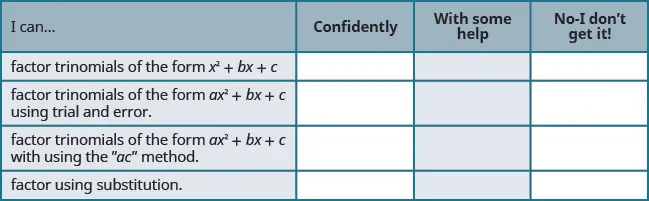After reviewing this checklist, what will you do to become confident for all objectives?

Order a print copy

As an Amazon Associate we earn from qualifying purchases.